Latest Banking jobs   »   Reasoning Ability Quiz For IBPS Clerk...

# Reasoning Ability Quiz For IBPS Clerk Prelims 2021- 16th August

Directions (1-5): Study the information carefully and answer the questions given below.
Eight people P, Q, R, S, T, U, V, and W are going on holiday on two different dates i.e. 5 and 8 in four different months i.e. January, March, April and May but not necessarily in the same order.
V goes on the 5th of April. Two persons are going in between V and P. More than two persons are going between U and S. U goes just after R but not in the same month. More than Four persons are going between S and W. None of them goes in the month that has an even number of days. More than one person goes between W and Q. T does not go in March.

Q1. Who among the following person goes on 8th march?
(a) U
(b) Q
(c) R
(d) T
(e) None of these

Q2. How many persons are going between T and S?
(a) Four
(b) One
(c) Two
(d) None
(e) Three

Q3. Who among the following person goes just before V?
(a) T
(b) R
(c) W
(d) U
(e) None of these

Q4. Four of the following five are alike in a certain and form a group, find the one that does not belong to that group?
(a) R
(b) Q
(c) U
(d) T
(e) P

Q5. Which of the following pair of persons goes in May?
(a) W, P
(b) U, W
(c) S, P
(d) U, S
(e) None of these

Direction (6-10): In these questions, relationship between different elements is show in the statements. The statements are followed by conclusions. Study the conclusions based on the given statements and select the appropriate answer:
(a) If only conclusion I follows.
(b) If only conclusion II follows.
(c) If either conclusion I or II follows
(d) If neither conclusion I nor II follows.
(e) If both conclusions I and II follow.

Q6. Statements: K<I>L≥Y>T=R<W≤D
Conclusion I: L>R II: D≥T

Q7. Statements: E=D≥C>V<U<N>M
Conclusion I: C<E II: N>D

Q8. Statements: O<Z=Q=T≥L≥A ≤B
Conclusion I: A<Z II: Z=A

Q9. Statements: P<H<J≤K>B>S≤X
Conclusion I: K≥P II: S>K

Q10. Statements: W≤ C=Y≥N>F=J≤T
Conclusion I: N≥T II: C>J

Directions (11-15): Study the following information carefully and answer the questions given below.
In a certain code language,
‘masked peace night property’ is coded as ‘la ja ta sa’,
‘senior peace property’ is coded as ‘sa ty ta’.
‘masked peace science wing’ is coded as ‘ja sa op nm’
‘water save wing’ is coded as ‘op vs rt’

Q11. Which of the following represents the code for ‘night’?
(a) sa
(b) ta
(c) la
(d) ja
(e) None of these

Q12. How is ‘peace’ coded in the given language?
(a) ja
(b) sa
(c) op
(d) nm
(e) Cannot be determined

Q13. How is ‘property’ coded in the given language?
(a) la
(b) ja
(c) sa
(d) ta
(e) Cannot be determined

Q14. What can be the code for ‘senior wing science’?
(a) op ja nm
(b) op ta nm
(c) ty nm op
(d) sa ta op
(e) Cannot be determined

Q15. How is ‘water’ coded in the given language?
(a) vs
(b) rt
(c) op
(d) nm
(e) Cannot be determined

Solutions

Solutions (1-5):
Sol.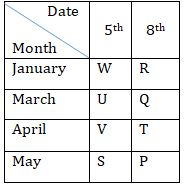S1.Ans(b)
S2.Ans(d)
S3.Ans(e)
S4.Ans(c)
S5.Ans(c)

Solution(6-10):
S6. Ans(a)
Sol. I: L>R(True) II: D≥T (False)

S7. Ans(d)
Sol. I: C<E(false) II: N>D (False)

S8. Ans(c)
Sol. I: A<Z(False) II: Z=A (False)

S9. Ans(d)
Sol. I: K≥P(False) II: S>K (False)

S10. Ans(b)
Sol. I: N≥T (False) II: C>J (True)

Solutions (11-15):
Sol.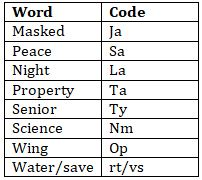S11.Ans(c)
S12.Ans(b)
S13.Ans(d)
S14.Ans(c)
S15.Ans(e)#### Congratulations!Incorrect details? Fill the form again here

•Reasoning Ability Quiz For IBPS Clerk Pr...
•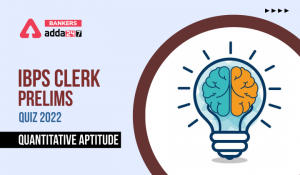Quantitative Aptitude Quiz For IBPS Cler...
•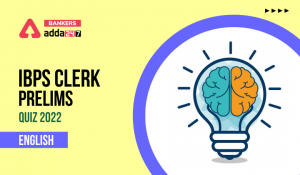English Quizzes For IBPS Clerk Prelims 2...
•Reasoning Ability Quiz For IBPS RRB PO P...
•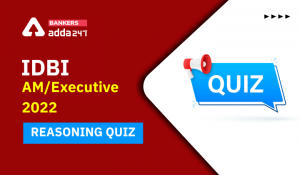Reasoning Ability Quiz For IDBI AM/Execu...
•Reasoning Ability Quiz For IBPS RRB PO P...